#Untitled Document 1. In presentation of classical physics; the light moved instantaneously. Ether was considered to be the transmitter. After special theory of Relativity appeared, which connected together time and space, ether was forgotten. But the history repeats . When Edwin Hubble discovered the expansion of the Universe, which does not just expand, but accelerates itself, a strange substance, appeared, not coming into interaction with anything, even with itself. Its only display is the deformation of the Universe. Thus its combined mass must include 96% of the mass of the Universe. The dark energy and the dark matter. Can there be any other reasons for the expansion of the Universe? And how does it really happen? 2. Theory of interaction 2.1. My suppositions are based on the special theory of Relativity, to be more exact on the contradiction laid in its basis. In the basis of special theory of Relativity, there is the principle of Relativity, according to which if body A moves rectilinearly forward without acceleration and rotation in isolated space towards body B at a speed V , with the same right, it is possible to consider, that body B moves towards body A with the speed V . V A = V B And conclusion is that only particles, which the mass of rest equals to zero, can move with the velocity of light. Depending on the movement speed longitudinal sizes get shorter, inertial mass increases and time slows in correlation :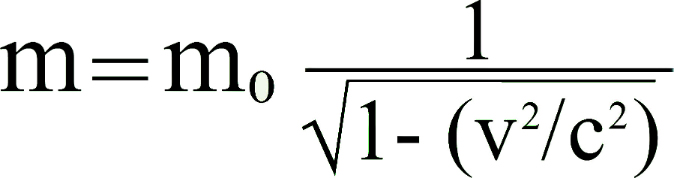And if to put the Earth instead of mass A and photon instead of mass B in the principle of relativity It will turn out, then if the photon moves in relation to the Earth at the velocity of Light Vγ = C , then the Earth in relation to the photon moves at the velocity of Light.It is not just a contradiction, they are mutually exclusive concepts. However, the theory of relativity works has been confirmed experimentally, is used in practice, what is wrong? 2.2. Let ' s conduct a mental experiment . Let's introduce the concepts “ real observer ” and “ conventional observer ” where the Real observer is man; and the conventional observer is his virtual copy. Then let's change the mass and the sizes of Conventional observer proportionally so that the dimensions of atom for him were proportional to the size of the solar system for the real observer. An electron revolves at such an enormous speed, that if a Real Observer were able to look at him, we would see a cloud instead of electron. But for diminished Conventional Observer the time will accelerate accordingly, all surrounding processes will slow down, and the nucleus will move away from electron so far that electron will revolve around the nucleus approximately at such a speed at which the Earth revolves around the Sun on the part of the Real Observer. 2.3. Will the time and the distance for Conventional Observer really change? And if so, how far? Let's equip the both observers with tools: A ruler and a watch. And if with the check of changing sizes everything is relatively simple, it is obvious, that the ruler will diminish l / l′ times according to it.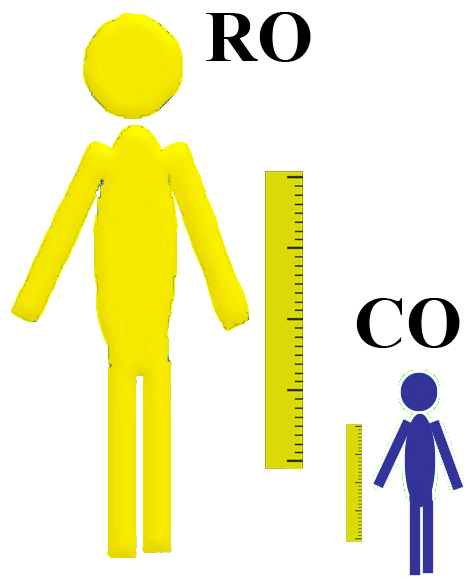There are some problems with the diminishing of mass of the watch. To model the change of the time according to the mass can be done simply by diminishing the mass of pendulum of metronome, thus the movement of metronome will increase. But for the purity of the experiment, it is necessary to change proportionally the mass of all watches on the whole. Let's use the example from Special Theory of Relativity. 2.4. If to draw a chart of dependence of mass, sizes and the time from the speed of motion, the chart will be as follows: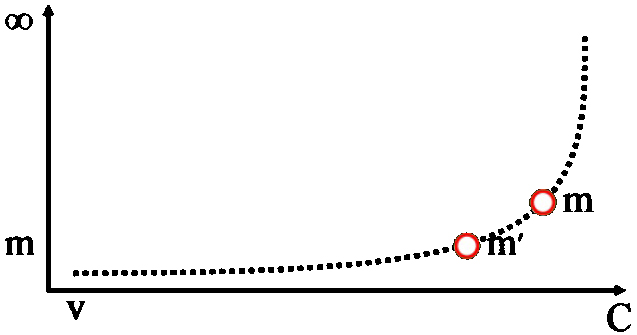Let's take two arbitrary points m and m′ stroke correlation between points is expressed as well as a simple correlation of mass m / m′. Longitudinal dimensions will get shorter l / l′, and time will slow down t / t′ likewise. m / m′ = l / l′ = t / t′ But in fact, any event for any observer will have the same amount of millimeters and will last for the same amount of seconds. What will change ? The duration of a second and the extent of a millimeter will be changed. All processes are changed for bodies, moving in inertial system, in other words, any watch will slow down its motion with the increase of inertial mass. On the part of observer, if to ignore the gravity, all internal processes will flow identically for bodies with different mass being in the space-hold in relation to each other. 2.5. In the system of two physical bodies with different mass, for example, the Moon and asteroid. A half-decay of the same element will take place with the identical periodicity. That's why the direct experiment on diminishing a watch is possible to do only with a watch, working on the principle of the interaction of masses, i.e. with a mechanical watch. 2.6. Time and space can't exist separately from the mass. Let's imagine an isolated space, where there is nothing. In such case time and distance are indefinable. If to place a point indivisible physical body with the mass of infinitely close to zero in the same space, time and distance will still be indefinable. If we place the second body, the distances will appear, but time is still indefinable. Time will appear only if bodies move towards each other. Motion in its turn automatically causes the change of mass. And if a close connection exists between mass and temporally-spatial co-ordinates, so we can conclude that for two bodies with different masses the co-ordinates will be different. 3. Conclusions 3 .1. If only longitudinal dimensions change in the inertial system, for two physical bodies, where the mass of the body A is more than ( m А > m В ) the mass of the body B , all the dimensions and distances for body A will be smaller and time will be slow than for the mass B m А / m В times.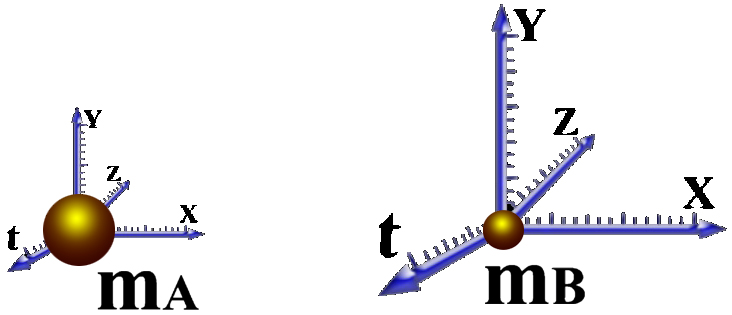The mass can be considered as a temporally-spatial equivalent. The temporally-spatial coefficient equals: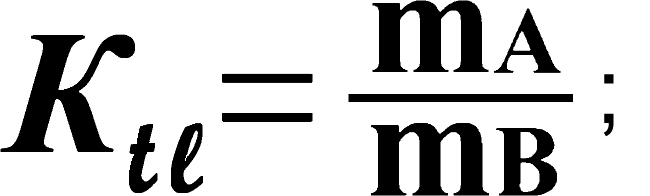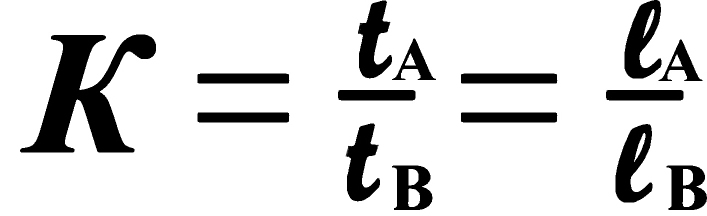3. 2. Thus, the principle of relativity will be just only for physical bodies with the same mass ( m A = m B ). For moving bodies with different mass in inertial system, the correlation will be as follows: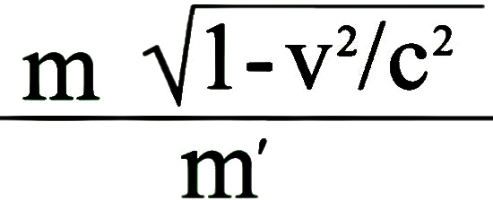3. 3. The principle of Interaction . If in isolated space the mass of the body A increases proportionally according to the mass of the body B m A / m B times, thus, with the same right, we can consider that the mass of the body B diminishes according to the body A m A / m B times. If to neglect the effect of gravity, proportional change of the mass of the body B towards body A , is equal to the movement of the bodies towards each other at a speed :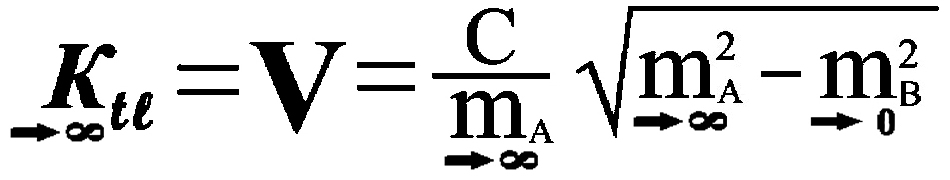4. If to compare these conclusions with the big bang theory, according to which just after the bang there was only energy, from which matter was formed later, thus the universe can be expanded. Let's imagine the system of observer and physical body in the position of rest towards each other. If the mass of a physical body increases, but not by moving of the mass from one place to another.The mass of capacity A has increased but thus the general number of the mass for the observer has remained the same. Namely, at the cause of the appearance of new mass in isolated space concerning observer, the mass of the observer will remain steady, it will diminish concerning the increasing mass of the body, then from the point of view of observer, the distance between the observer and the body will increase accordingly m/m′ . At close distances the gravity will predominate. But with the increasing of the distance (e.g. in the case of forming galaxy) gravity weakens and the effect of mutual interaction remains unchangeable. That's why the farther the increasing galaxy is, the weaker gravity is, the space will expand quicker, and the galaxy will move away. But no matter how far we look into space, we see practically formed galaxies, the mass of which doesn't increase. That's why the reason of the Universe expansion is the fact that is behind the scene of events. Behind the invisible bodyline after which everything moves faster than the light. There behind the horizon, the echo of the act of the formation of the matter reaches us, which takes place at billion years from us, reforming the energy into the mass. The mass of the observer or the tool for monitoring, remaining steady, diminishes according to increase of combined supernova matter. It brings to the expansion of space, which we observe how galaxies are fluttering with acceleration to different sides from us. As the reason of the expansion is relative diminishing of the mass of the observer, the observer will always be in the center of expansion , no matter, what point of the visible space he might occupy. The symmetry lies in the fact that if we could what is the happening from the point of view of the supernova mass from the opposite side of the horizon, everything could happen quite on the contrary. We would see that behind the horizon everything including the Earth, moves at the velocity of light irrespective on the primary speed. We can suppose that for the real observer there is an analogue of the horizon of the events in the micro world on the atomic level, The Internal Horizon. This is an invisible line behind which everything moves at the velocity of light irrespective of the primary speed. 4. If the observer proportionally diminishes as to the source of the light, the specter as to the observer will gradually move to the side of the red until it turns into the radio waves. The white noise is nothing but the light of the newer stars, which are close to the horizon of events. 5. Theoretically, for distant travelling it is enough to increase the mass of the spaceship and all the distances will get shorter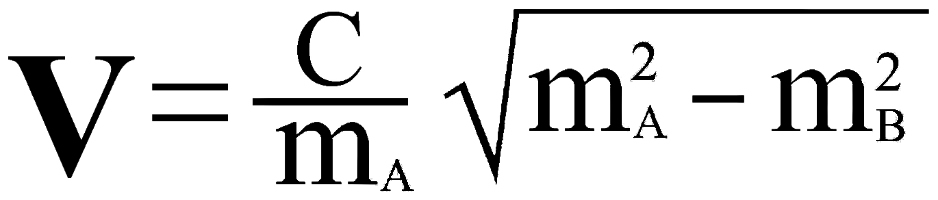тimes. My theory does not reject the existence of dark matter. I only assert, that, if dark matter exists, it must expand together with the Universe. Having been equipped with the theory, there is a chance to make an unbelievable technological breakthrough. But it's quite a different story.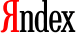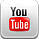This free website was made using Yola.

No HTML skills required. Build your website in minutes.